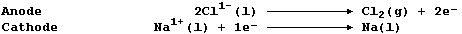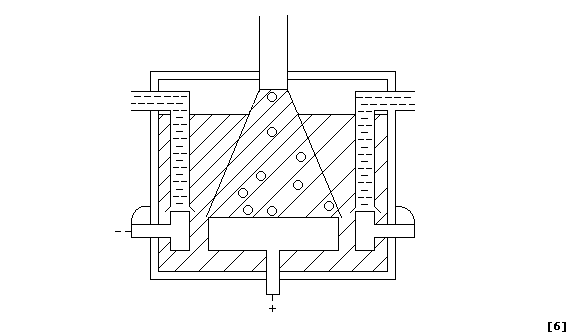```METALS: EXTRACTION of SODIUM
Sodium, the fourth most abundant metal in the Earth's crust (2.4%), is
usually found as the chloride (e.g., in the ore rock salt or dissolved
in sea water). Sodium has a low melting point (98°C) and a low density
(0.97 g cm-ł), which are characteristics of Group 1 metals, and forms
(usually) white or colourless compounds in only one oxidation state
(I), which is typical of main group metals.
[.. K > Ca > Na > Mg > Al > Zn > Fe > Sn > Pb > (H) > Cu > Hg > Ag ..]
1.  Sodium is extracted by the electrolytic reduction of purified,
molten rock salt; the ionic equations for the reactions occurring at
the electrodes are:``````(a) Label this diagram of a Downs electrolytic cell with: Carbon anode;
Chlorine gas; Insulator; Molten electrolyte; Molten sodium; and, Steel
cathode.``````(b) To lower the melting point of sodium chloride from 774°C to about
600°C, and so reduce the energy costs, calcium chloride is added to the
rock salt. As a result of this added impurity, a mixture of molten
sodium and solid calcium is obtained. Write an ionic equation for the
reduction of calcium ions at the cathode.
_______________________________________________________________________

(c) Water must not be present in the Downs cell, partly because a
different cathodic reaction occurs. Thus, hydrogen gas is evolved at
the cathode from the electrolysis of an aqueous solution containing

Na1+(aq), Cl1-(aq), H1+(aq), and OH1-(aq) ions. Write an ionic equation
for the reduction of hydrogen ions at the cathode.
_______________________________________________________________________

(d) After manufacture, sodium is stored under oil because it is rapidly
oxidized by atmospheric oxygen. Furthermore, sodium reacts explosively
with many other non-metallic elements and compounds. Construct the
symbol equation for the reaction of sodium with:
Difluorine ____________________________________________________________
Dioxygen ______________________________________________________________
Water _________________________________________________________________
```

```Sodium's high reactivity has necessarily restricted its use to a few
applications. Thus, aside from its incorporation into specialized
alloys (e.g., Na-Hg amalgam), it is used in street vapour lamps, as a
coolant in nuclear reactors, and as a chemical reducing agent.
2.  Sodium's use as a coolant in nuclear reactors depends on three of
the metal's properties. First, it is a liquid at temperatures between
98°C and 883°C, so the metal flows easily around the hot reactor core
(which operates at a temperature of about 660°C). Second, it is a good
thermal conductor (because of its free moving delocalized electrons),
so excess heat generated in the core is conducted away efficiently.
And third, it has a relatively high thermal capacity, so large amounts
of heat energy are absorbed for any given temperature rise.
[Q = n × z × F and Q = I × t, where: Q, measured in coulombs (C), is
the quantity of electricity; n is the number of moles of substance
evolved at the electrode; z is the charge on the ion; F is a constant,
with a value of 96500 C mol-ą; I, measured in amps (A), is the current;
and t, measured in seconds (s), is the time.]
(a) A fast-breeder reactor under construction required 690 tonnes of
sodium. A typical Downs electrolytic cell operates continuously at a
current of 25 kA. Determine the time (t) it would take to obtain the
required mass of sodium - as follows.
Convert this mass (m) of sodium from tonnes to grammes (where
1 tonne = 1000 kg). ___________________________________________________
Calculate the number of moles (n) in this mass (m) of sodium. _________
_______________________________________________________________________
Calculate the quantity of electricity (Q) required to deposit this
number of moles (n) of sodium at the cathode. _________________________
_______________________________________________________________________
And finally, calculate the time (t) taken for this quantity of
electricity (Q) to be used. ___________________________________________
_______________________________________________________________________

(b) The volume (V1) of one mole of any gas at room temperature (25°C =
298 K; T1) and pressure (0.1 MPa; P1) is 24 dmł; furthermore, the
following relationship holds true for gases:
P1 × V1     P2 × V2
ľľľľľ = ľľľľľ
T1         T2
Determine the volume (V2) of chlorine gas obtained, at room temperature
and high pressure (20 MPa; P2), during the production of this mass of
mass of sodium - as follows.
State the number of moles (n) of chlorine atoms, Cl(g), formed at the
anode. ________________________________________________________________
State the number of moles of chlorine gas, Cl2(g), evolved at the
anode. ________________________________________________________________
Calculate the volume of gas (V1) obtained at room temperature (T1) and
pressure (P1). ________________________________________________________
Then, using the above relationship, calculate the volume (V2) of gas
at the increased pressure (P2). _______________________________________
_______________________________________________________________________
_______________________________________________________________________
_______________________________________________________________________

3.  Sodium metal reduces liquid ammonia to sodium amide, NaNH2(s), a
sparingly used fertilizer. Construct the symbol equation for this redox
reaction, which is executed under anhydrous and anaerobic conditions.
_______________________________________________________________________

Dr. R. Peters                   Next                     Contents' List```